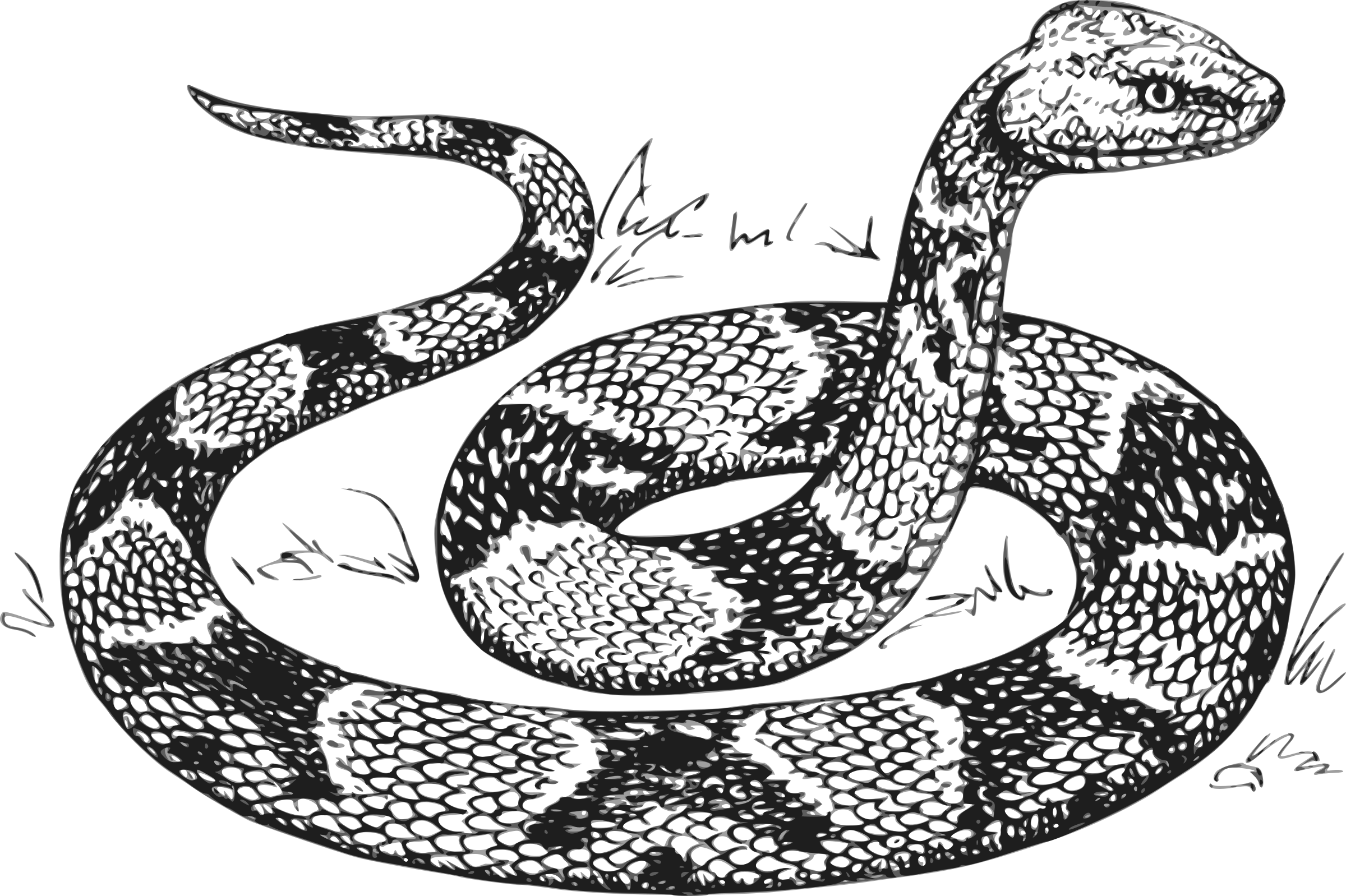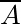# Mathematical Notation for Python Developers (Part 2)

## Mathematical Notation for Python Developers (Part 2) | Set NotationIn the previous article, we went through a basic understanding of what sets are and how their memberships work through simple Python 3 snippets.

In this part 2 we will keep on understanding more about set relationships using our friend Python 3.

### Union

As the name denotes, it is a union of 2 or more sets. Unions are denoted with the symbol ∪.

If A and B are sets and A = {1, 2, 3}, B={3, 4, 5} then A ∪ B = {1, 2, 3, 4, 5}

``````# For more info [https://www.w3schools.com/python/ref_set_union.asp](https://www.w3schools.com/python/ref_set_union.asp)

a = {1, 2, 3}
b = {3, 4, 5}

c = a.union(b)
print(c)
# >> {1, 2, 3, 4, 5}

"""
If you do not want to use any in-build functionalities you can simply use the | operator to unite sets.
"""

c = a | b

print(c)
# >> {1, 2, 3, 4, 5}``````

### Intersection

Intersections are used when you want to obtain the elements that exist in both sets, intersections are denoted with the symbol .

If A and B are sets and A = {1, 2, 3}, B={3, 4, 5} then A ∩ B = {3}

``````# For more info check
# [https://www.w3schools.com/python/ref_set_intersection.asp](https://www.w3schools.com/python/ref_set_intersection.asp)

a = {1, 2, 3}
b = {3, 4, 5}

c = a.intersection(b)
print(c)
# >> {3}

"""
If you do not want to use any in-build functionalities you can simply use the & operator to get the set intersection.
"""

c = a & b

print(c)
# >> {3}``````

### Difference

The difference is the set of all elements of A that are not elements of B. Difference is denoted with the symbol -.

If A and B are sets and A = {1, 2, 3}, B={3, 4, 5} then A - B = {1, 2}

``````# For more info check:
# [https://www.w3schools.com/python/ref_set_difference.asp](https://www.w3schools.com/python/ref_set_difference.asp)

a = {1, 2, 3}
b = {3, 4, 5}

c = a.difference(b)
print(c)
# >>  {1, 2}

"""
If you do not want to use any in-build functionalities you can simply use the - operator to get the difference.
"""

c = a - b

print(c)
# >>  {1, 2}``````

### Complement

If A is a set and A= {1, 2, 3} then, the complement of A will refer to the elements, not in A. Complement can be denoted in different ways like A’ or Aᶜ or:NOTE: In case you are wondering why the last complement notation is in image format, that is because medium does not render some latex configurations correctly.

Best Coding Languages to Learn in 2019 | Data Driven Investor During my years as an undergrad, I skipped many night-outs to pick up Java hoping it would one day help me get ahead in…www.datadriveninvestor.com

Let’s assume that set X contains all the possible numbers in the universe and set X= {1 ,2, 3, 4, 5, 6, 7} and we have another set denoted as set A, A = {1, 2, 3} then Aᶜ = {4, 5, 6, 7}.

I am not gonna represent this notation in Python 3 since it is similar to what the difference notation represents.

### Symmetric Difference

Symmetric Difference is the set that contains elements that exist in A and B but not both. Symmetric Difference is denoted by the symbol ∆.

If A and B are sets and A = {1, 2, 3}, B={3, 4, 5} then A — B = {1, 2, 4, 5}

``````# For more info check:
# [https://www.w3schools.com/python/ref_set_symmetric_difference.asp](https://www.w3schools.com/python/ref_set_symmetric_difference.asp)

a = {1, 2, 3}
b = {3, 4, 5}

c = a.difference(b)
print(c)
# >> {1, 2, 4, 5}

"""
If you do not want to use any in-build functionalities you can simply use the ^ operator to get the difference.
"""

c = a ^ b

print(c)
# >> {1, 2, 4, 5}``````

### Cartesian Product

Cartesian Product is the multiplication of two sets to form the set of all ordered pairs. Cartesian product is denoted by the multiplication symbol ×.

If A and B are sets and A = {1, 2, 3}, B={4,5,6}

A × B = {(a,b): a ∈ A and b ∈ B}

then A × B = {(2, 4), (3, 4), (1, 5), (1, 4), (2, 6), (3, 6), (1, 6), (2, 5), (3, 5)}

``````a = {1,2,3}
b = {4,5,6}
v = {(x, y) for x in a for y in b}

print(v)
# >> {(2, 4), (3, 4), (1, 5), (1, 4), (2, 6), (3, 6), (1, 6), (2,5), # (3, 5)}``````

The cartesian product is much harder to grasp in the beginning, so I will leave you a few links that might help you understand better what the cartesian product is about.

Cartesian Product For two sets A and B, the Cartesian product of A and B is denoted by A×B and defined as: A×B = { (a,b) | aϵA and bϵB }…www.mathstopia.net

### Power set

If A is a set, then the power set of A is the set of all subsets of A, this includes the empty set and A itself. Power set of A is denoted by P(A).

A = {1, 2, 3} then P(A) = {{},{1},{2},{3},{1,2},{1,3},{2,3},{1,2,3}}

NOTE:

In the Python snippet below you will notice that we are not using sets within sets. This happens because every object you add inside of a set must be immutable. So you will see the parent set and instead of child sets you will see itertools objects with the powerset iterations.

WELL, WHY IN THE HELL DO THEY TO BE IMMUTABLE YOU MIGHT ASK?

Because every object you add inside of a set is gonna be hashed, that is the way sets in Python to figure out the unique values and index them, meaning you cannot add sets, lists, dictionaries or any other mutable object within a set.

Hashable An object is hashable if it has a hash value which never changes during its lifetime (it needs a hash() method), and can be compared to other objects (it needs an eq() or cmp() method). Hashable objects which compare equal must have the same hash value. Hashability makes an object usable as a dictionary key and a set member, because these data structures use the hash value internally. All of Python’s immutable built-in objects are hashable, while no mutable containers (such as lists or dictionaries) are. Objects which are instances of user-defined classes are hashable by default; they all compare unequal, and their hash value is their id().

``````from itertools import combinations, chain

# Defines the parent set and adds an empty tuple inside (this is our # equivalent to an empty set)
empty_set = {(), }

def powerset(someset):

try:

# Check if someset is a set

someset.isdisjoint

except AttributeError:

raise TypeError(

f"{powerset.__name__} accepts only a set-like object as       parameter"

) from None

# Get the length/cardinality of the set.

combs =  set()

for k in range(1, size+1):
"""

Combinations are emitted in lexicographic sorted order. So,if      the input iterable is sorted, the combination tuples will be    produced in sorted order.

"""

subset = combinations(someset, k)

"""

Make an iterator that returns elements from the first iterable  until it is exhausted, then proceeds to the next iterable, until all of the iterables are exhausted. Used for treating consecutive sequences as a single sequence.

"""

return set(chain(empty_set, *combs))

print(powerset({1, 2, 3}))
# >> {(1, 3), (2,), (1, 2), (1, 2, 3), (2, 3), (1,), (), (3,)}``````

### Cardinality

Cardinality represents the number of elements within a set also known as set length. If A is a set then the cardinality of A is denoted by |A|.

If A is a set and A = {1, 2, 3} then |A| = 3

``````a = {1, 2, 3}
cardinality = len(a)
print(cardinality)
# >> 3``````

https://www.rapidtables.com/math/symbols/BasicMathSymbols.html